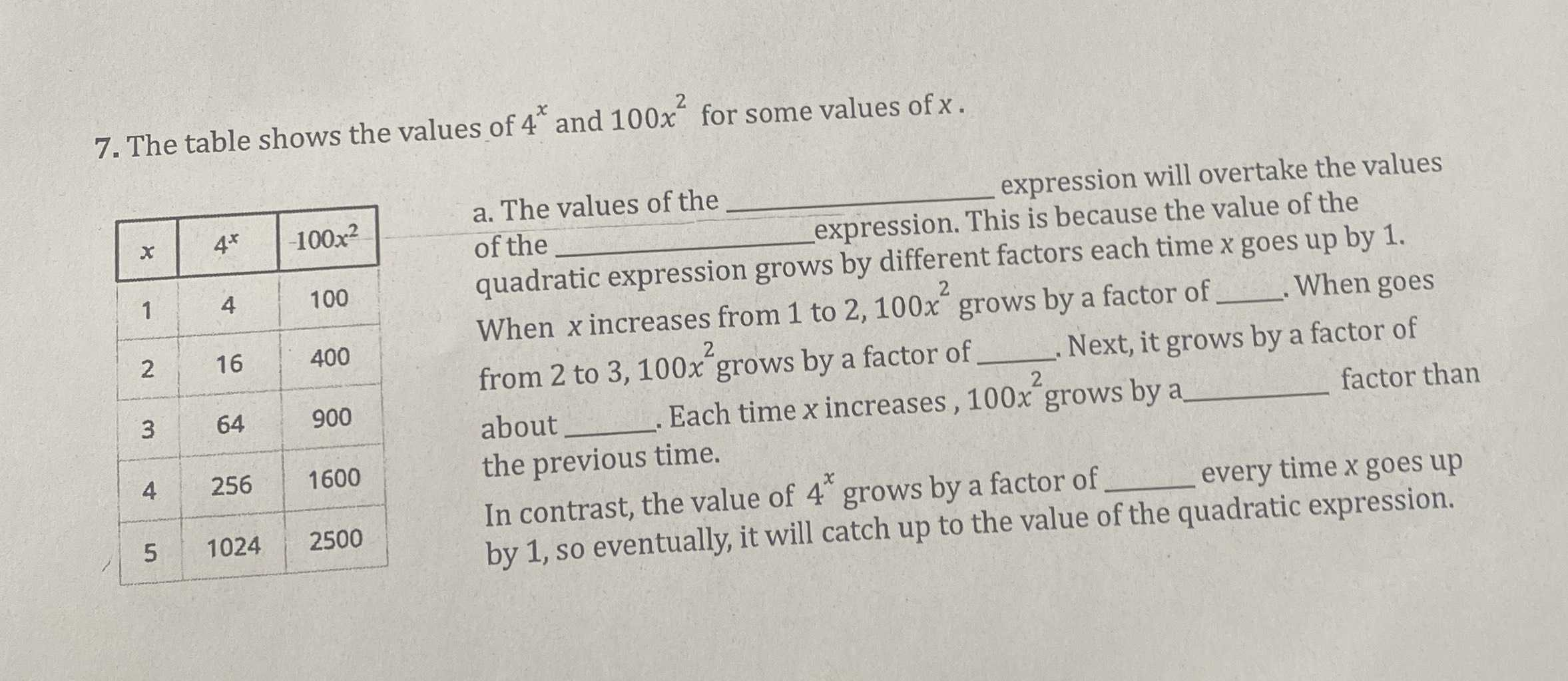### ¿Todavía tienes preguntas de matemáticas?

Pregunte a nuestros tutores expertos
Algebra
Pregunta7. The table shows the values of $$4 ^ { x }$$ and $$100 x ^ { 2 }$$ for some values of $$x$$ .

a. The values of the of the quadratic expression grows by different factors each time $$x$$ goes up by $$1$$ . When $$x$$ increases from $$1$$ to $$2,100 x ^ { 2 }$$ grows by a factor of from $$2$$ to $$3,100 x ^ { 2 }$$ grows by a factor of When goes about the previous time. In contrast, the value of $$4 ^ { x }$$ grows by a factor of a factor of by $$1$$ , so eventually, it will catch up to the value of the quadratic expression.

a) 4$$^x$$ , 100x$$^2$$